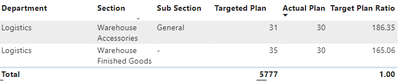cancel
Showing results for
Did you mean:Anonymous
Not applicable

## How can i show the ratio of the Actual Plan?In the above image, I have calculated the Target Plan ratio using this line of code.

DAX Formula:

Target Plan Ratio =
VAR _total =
SUMX( ALL( 'MJK' ), [Plan Listed Headcount Of Employee] )
VAR _subtotal =
SUM( 'MJK'[Plan Listed Headcount Of Employee] )
RETURN
DIVIDE( _total, _subtotal )

However, for the calculation of Actual plan ratio, i am not able to calculate because my actual plan column is a calculated measure. using this line of code.

MJK Employee Count (Actual) =
COUNTROWS(
FILTER(
ALL( 'MJK Total Employees' ),
'MJK Total Employees'[Department]
= SELECTEDVALUE( 'MJK'[Department] )
&& 'MJK Total Employees'[Section]
= SELECTEDVALUE('MJK'[Section ])
&& 'MJK Total Employees'[Sub Section]
= SELECTEDVALUE( 'MJK'[Sub Section] )
)
)

For eg. How i get the 186.35? It is calculated as 5777/31 =186.35

In this case, the total actual plan is 4145 and i want it to calculate as 4145/30 = 138.16.

However, the total actual column is a calculated measure as described above, therefore, i cant use the same DAX function to test it out like how i did the Target Plan Ratio.

Therefore, any way to calculate the actual ratio even if it is a calculated measure? Any help is appreciated. Thanks.

9 REPLIES 9Anonymous
Not applicable

Any help?Community Support

Hi @Anonymous ,

According to your description, are you saying that the total number of statistics created for the measure [actual plan] is 4145, but in the final calculation, the actual total number used is 5777 corresponding to the Target plan field, is that what you mean?

Also what is your data model, if you can provide test data (remove sensitive information), I will answer for you as soon as possible.

How to Get Your Question Answered Quickly - Microsoft Power BI Community

Best Regards,
HenryAnonymous
Not applicableAnonymous
Not applicable

I have shared my PBI report previously, but it cant be opened as other users have requested before.

Nonetheless, the 5777 is the total plan value. In this case, the total actual is 4145 but it is not shown in the table using the above code. What i want now is to calculate ratio for total actual column, is there a way to do it?Community Support

Hi @Anonymous ,

For your description, it should be a problem that MEASURE does not show the correct calculation results. You can refer to the following similar solutions and related blogs, hope it will help you.

Solved: Re: Sum of values in a measure with divide measure - Microsoft Power BI Community

How to Make Measures Total Correctly in Power BI Tables - ArcherPoint

Best Regards,
Henry

If this post helps, then please consider Accept it as the solution to help the other members find it more quickly.Anonymous
Not applicable

The calculation is exactly what i want, but it is only not showing the total. Unless you have a better way of calculating it, i am open to try and test it on my side.

The main point is whether can i create a ratio for based on the actual column, and in this case, my actual column is a calculated measure which is why i have tried several ways and i still cant resolve them.Super User

@Anonymous , Create a measure for GT

sumx(addcolumns(summarize (all('MJK Total Employees'), 'MJK Total Employees'[Department], 'MJK Total Employees'[Section] ), "_sum", [MJK Employee Count (Actual) ] ), [_sum])

then you can try % or total

sumx(addcolumns(summarize (allselected('MJK Total Employees'), 'MJK Total Employees'[Department], 'MJK Total Employees'[Section] ), "_sum", [MJK Employee Count (Actual) ] ), [_sum])

then you can try % or total

If this does not help
Can you share sample data and sample output in table format? Or a sample pbix after removing sensitive data.Anonymous
Not applicable

Sorry, I have tested your DAX and it is not what i want.

For eg. How i get the 186.35? It is calculated as 5777/31 =186.35

In this case, the total actual plan is 4145 and i want it to calculate as 4145/30 = 138.16.

However, the total actual column is a calculated measure as described above, therefore, i cant use the same DAX function to test it out like how i did the Target Plan Ratio.Anonymous
Not applicableAnnouncements#### Exclusive opportunity for Women!

Join us for a free, hands-on Microsoft workshop led by women trainers for women where you will learn how to build a Dashboard in a Day!#### Power Platform Conference-Power BI and Fabric Sessions

Join us Oct 1 - 6 in Las Vegas for the Microsoft Power Platform Conference.Top Solution Authors
Top Kudoed Authors
Users online (2,849)